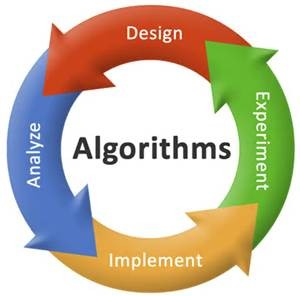1. Define the concept of an algorithm.

An algorithm is any well-defined computational procedure that takes some value (or set of values) as input and produces some value (or set of values) as output. In short, it can be seen as a sequence of computational steps that transform the input into the output.
2.What are the arguments present in pattern matching algorithms?

These are the following arguments which are present in pattern matching Algorithms.
1) Subject,
2) Pattern
3) Cursor
4) MATCH_STR
5) REPLACE_STR
6) REPLACE_FLAG

3. Explain the function SUB in algorithmic notation?

In the algorithmic notation rather than using special marker symbols, generally people use the cursor position plus a substring length to isolate a substring. The name of the function is SUB.
SUB returns a value the sub string of SUBJECT that is specified by the parameters i and j and an assumed value of j.

4. In Algorithmic context how would you define book keeping operations?

Usually when a user wants to estimate time he isolates the specific function and brands it as active operation. The other operations in the algorithm, the assignments, the manipulations of the index and the accessing of a value in the vector, occur no more often than the addition of vector values. These operations are collectively called as book keeping operations.

5. Define and describe an iterative process with general steps of flow chart?

There are four parts in the iterative process they are
Initialization: -The decision parameter is used to determine when to exit from the loop.
Decision: -The decision parameter is used to determine whether to remain in the loop or not.
Computation: – The required computation is performed in this part.
Update: – The decision parameter is updated and a transfer to the next iteration results.

6. State recursion and its different types?

Recursion is the name given to the technique of defining a set or a process in terms of itself. There are essentially two types of recursion. The first type concerns recursively defined function and the second type of recursion is the recursive use of a procedure.

7. Define and state the importance of sub algorithm in computation and its relation ship with main algorithm?

A sub algorithm is an independent component of an algorithm and for this reason is defined separately from the main algorithm. The purpose of a sub algorithm is to perform some computation when required, under control of the main algorithm. This computation may be performed on zero or more parameters passed by the calling routine.

8. Name any three skills which are very important in order to work with generating functions.

The three most important skills which are used extensively while working with generating functions are
1)Manipulate summation expressions and their indices.
2)Solve algebraic equations and manipulate algebraic expressions, including partial function decompositions.
3)Identify sequences with their generating functions

9. What is the general strategy for Markov Algorithm?

The general strategy in a Markov Algorithm is to take as input a string x and, through a number of steps in the algorithm, transform x to an output string y. this transformation process is generally performed in computers for text editing or program compilation.

10. Define string in an algorithmic notation and an example to support it?

In the algorithmic notation, a string is expressed as any sequence of characters enclosed in single quote marks.